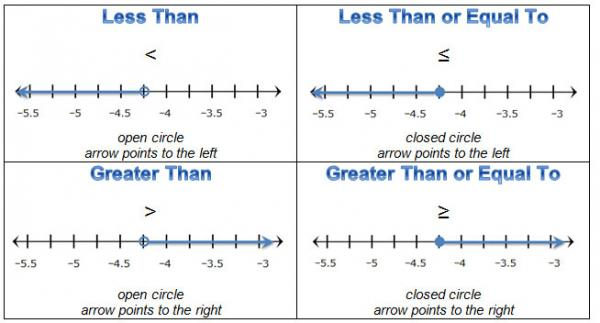Let's Get Started

We're going to learn how to solve inequalities with one variable using number lines and symbolic representations to express the solution.

TEKS Standards and Student Expectations

A(5) Linear functions, equations, and inequalities. The student applies the mathematical process standards to solve, with and without technology, linear equations and evaluate the reasonableness of their solutions. The student is expected to:

A(5)(B) solve linear inequalities in one variable, including those for which the application of the distributive property is necessary and for which variables are included on both sides

Resource Objective(s)

Students will solve one-variable inequalities using a variety of representations, including tables, graphs, and symbolic representations.

Essential Questions

How can a number line be used to represent the solutions to an inequality?

How can a table of values be used to represent the solutions to an inequality?

Vocabulary

Introduction

A number line is a useful tool for representing numbers. Solutions to an equation can be represented using points on the number line. For example, the solution to the equation 10x = 90 is x = 9. This solution can be clearly represented on a number line by plotting the point.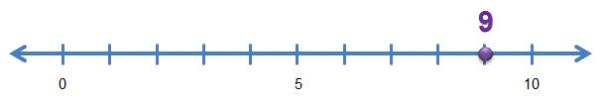Inequalities have a range of solutions. Number lines are also useful to represent these range of solutions that you would see in an inequality.

Example

Anu has a bus pass worth $25. Each bus ride costs her$2.50. What are some possible values for the number of bus rides (n) that Anu can take in one month?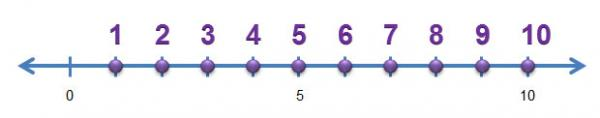The number line can be used to represent the whole numbers between 0 and 10, which are possible solutions for the inequality, 2.50n ≤ 25. This inequality represents the relationship between the cost per bus ride, the number of bus rides, and the amount of money used on the bus pass.

Solving One-Variable Inequalities Using Tables

One way that you can solve one-variable inequalities is to use a graph or a table.

Example

Concert tickets cost $35.50 each, plus a one time$12 convenience fee. A Spanish Club has a budget of \$438 to spend on concert tickets. How many concert tickets (x) can the Spanish Club purchase?

First write an inequality that could be used to determine possible values of x for this situation.

Next use a graphing calculator or online graphing calculator to generate a table of values for the equation Y1 = 35.50x + 12 and the equation Y2 = 438. Use the information from your graphing calculator to complete the table in your OnTRACK Algebra Journal.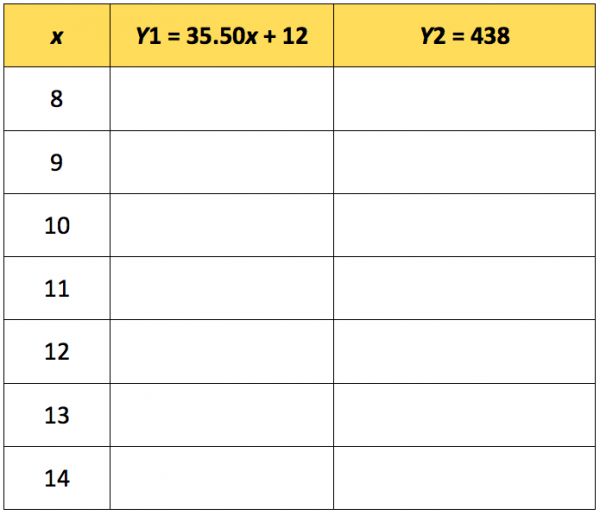To use the online graphing calculator follow the steps below:

1. Select Graphing Calculator mode.
2. Enter 35.50x + 12 for equation 1.
3. Enter 438 for equation 2.
4. Select Table.
5. Select an equation.
6. Set the x-value to begin at 8.
7. Set the interval to 1.

Solving One-Variable Inequalities Using Symbolic Representations

Let’s practice how you can apply properties of real numbers, including the commutative property, the associative property, and the distributive property, to solve an inequality that is written in symbolic form. For inequalities, properties of algebra apply just like they do with equations.

When solving an inequality where you have multiplied or divided by a negative number you have to make sure that the inequality symbol still creates a true statement.

Complete the table below in your OnTRACK Algebra Journal. Make sure to include the appropriate inequality symbol. The first row has been completed for you.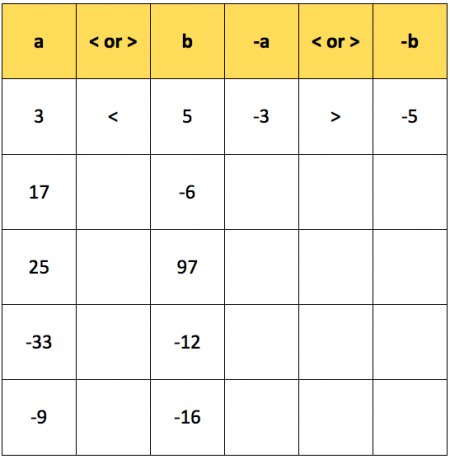Remember, whenever a linear inequality is multiplied or divided by a negative number, the inequality symbol must be reversed in order to preserve the value of the inequality. Practice this concept with the following three questions.

Representing Solutions of Inequalities on Number Lines

You can also represent solutions to one-variable inequalities using a number line.

There is one number that serves as a boundary point with any inequality. Sometimes that boundary point is included in the solution to the inequality and sometimes it is not.

The boundary point should be included in the solution when the inequality is less than or equal to (≤) or greater than or equal to (≥). This is represented by a closed circle on the boundary point.

The boundary point is not included when the inequality is less than (<) or greater than (>)This is represented by an open circle on the boundary point.

The arrow goes to the right when the inequality symbol is greater than (>) or greater than or equal to (≥).

The arrow goes to the left when the inequality symbol is less than (<) or less than or equal to (≤).

Summary

Many real-world problems require inequalities in order to solve them. You will also encounter inequalities as you continue your study of algebra in high school and in college. It is important to be able to solve one-variable inequalities for a variety of reasons. The table below summarizes the important points to remember when solving problems with inequalities.